Engineering Jobs   »   Civil Engineering quizs   »   NHPC-JE

# NHPC-JE’21 CE: Daily Practices Quiz. 24-Sep-2021

Know your strengths and practice your concepts with this quiz on NHPC JE Recruitment 2021. This quiz for NHPC JE Recruitment 2021 is designed specially according to NHPC Syllabus 2021.

Quiz: Civil Engineering
Exam: NHPC-JE
Topic: Miscellaneous

Each question carries 1 mark
Negative marking: 1/4 mark
Time: 8 Minutes

Q1. In order to draw parallel lines, concentric circles and parallel curves, command used in AutoCAD is:
(a) Array
(b) Parallel
(c) Copy
(d) Offset

Q2. Calculate the total correction (m) required, if a line measured using 20 m chain is 2380 m. actual length of the chain is 20.2 m.
(a) 22.6
(b) 23.56
(c) 23.8
(d) 476

Q3. Dickens formula predicts maximum flood discharge, Q, in terms of the area, A, and the coefficient, c, as Q = cA^n. The value of n is
(a) 0.25
(b) 0.50
(c) 0.67
(d) 0.75

Q4. The ‘Center Line Method’ is specially adopted for estimating-
(a) Polygonal Buildings only
(b) Circular, hexagonal and other geometric
(c) Circular buildings only
(d) Hexagonal buildings only

Q5. Which of the following is/are the properties of good quality of stones?
A. Crushing strength greater than 1000 kg/〖cm〗^2
B. Specific gravity should be greater than 2.6
C. Stones should be well seasoned before putting into use
(a) A, B and C
(b) Only B
(c) B and C
(d) Only A

Q6. What is the surface area (m²) of setting tank used for design discharge of 1.5 m³/s? (Assume design overflow rate of tank as 40 m³/m²/day)
(a) 2045.32
(b) 3240.44
(c) 4525.33
(d) 5076.13

Solutions

S1. Ans.(d)
Sol. Offset command is used in AUTO CAD in order to draw parallel lines, concentric circles and parallel curve. While array command is used to repeat the draw pattern and objects in Auto CAD.

S2. Ans.(c)
Sol. True length of chain (l) = 20 m.
Actual length of chain (l) = 20.2m.
Measured length of line (L’) = 2380m.
Total correction required (C) =?
C=L^’ ((l^’-l)/l)
=2380((20.2-20)/20)
=2380×0.01
=23.8 m.

S3. Ans.(d)
Sol. Dickens formula
▭(Q=CA^(3/4) )
▭(n=3/4)

S4. Ans.(b)
Sol. Center line method→ This method is suitable for symmetrical cross-sections like circular, hexagonal and other geometric shapes. This method is suitable for walls have the same thickness. In this method the center line for each type worked out and then multiplied by the breadth and depth of respective item to get the total quantity.

S5. Ans.(a)
Sol. There are some following property of good building stone-
– The crushing strength of good building stone should not be less than 100 MPa or 1000 kg/〖cm〗^2
– The specific gravity should be greater than 2.6.
– Stone should be well seasoned before putting into use.
– Stone should not absorb more than 5% water by its dry weight.
– The hardness of stone should be greater than 17.

S6. Ans.(b)
Sol. Given
Discharge (Q) = 1.5 m³/sec.
Overflow rate (V_o) = 40 m³/m²/day
Surface Area (m²) =?
Overflow rate (V_o) = Q/(B.L)
40 = 1.5/(B.L)
Surface Area (B.L) = (1.5×24×60×60(m^3 \/day.))/40(m^3 \/m^2 \/day)
= 3240 m²

Sharing is caring!

Thank You, Your details have been submitted we will get back to you.
•NHPC Result Through GATE 2021, Download ...
•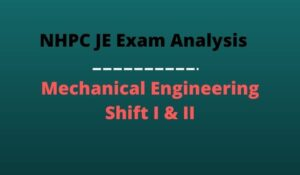NHPC JE Mechanical Exam Analysis 2022 Di...
•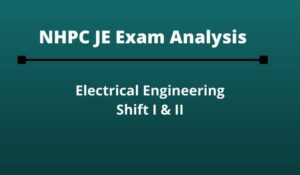NHPC JE Exam Analysis 2022 Electrical En...
•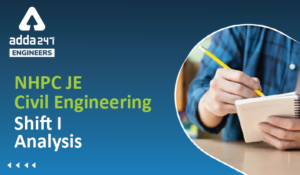NHPC JE Exam Analysis Civil Engineering ...
•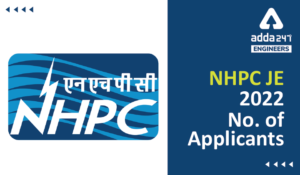NHPC JE Application Form 2022, Check No....
•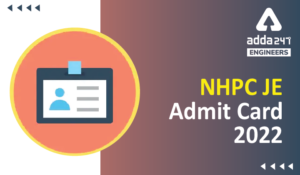NHPC Junior Engineer Admit Card 2022, Di...